• September 23, 2020, 03:28:41 PM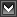• Welcome, Guest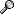### News:

This Forum Beta is ONLY for registered owners of D-Link products in the USA for which we have created boards at this time.

Pages: 

###AuthorTopic: Do I need to worry about these...  (Read 34482 times)

#### Yanta

• Level 2 Member
•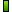• Posts: 66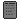##### Do I need to worry about these...
« on: August 26, 2012, 02:19:44 AM »

Aug 26 16:43:50 user alert kernel: IntrusionXXX -> IN=ppp1 OUT= MAC=
Aug 26 16:53:59 user alert kernel: IntrusionXXX -> IN=ppp1 OUT= MAC=
Aug 26 16:55:57 user emerg kernel: IP Spoofing -> IN=ppp1 OUT= MAC=
Aug 26 17:03:50 user alert kernel: IntrusionXXX -> IN=ppp1 OUT= MAC=
Aug 26 17:14:17 user alert kernel: IntrusionXXX -> IN=ppp1 OUT= MAC=
Aug 26 17:23:53 user alert kernel: IntrusionXXX -> IN=ppp1 OUT= MAC=
Aug 26 17:33:48 user alert kernel: IntrusionXXX -> IN=ppp1 OUT= MAC=Logged

#### FurryNutz

• Poweruser
•   ▲
▲ ▲
•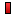• Posts: 49205
•##### Re: Do I need to worry about these...
« Reply #1 on: August 27, 2012, 07:37:08 AM »

Might be just there way of reporting that the modem has blocked something. I might contact your local regional DLink support office and ask.Logged
"Nothing Funny about It...." We are not here to Impress anyone! You have a be a COMPETENT user first to under stand COMPETENT help!

#### Yanta

• Level 2 Member
•• Posts: 66##### Re: Do I need to worry about these...
« Reply #2 on: August 27, 2012, 04:50:00 PM »

Might be just there way of reporting that the modem has blocked something. I might contact your local regional DLink support office and ask.

Th entire conversation went like this...

"These are just log messages. Even if the status says 'emergency' it is just telling you something has been blocked"

"as long as your firewall in the router is turned on your router and network cannot be hacked. Thank you for calling Dlink".

The technician was unable to identify what IP spoofing or IntrusionXXX meant.Logged

#### FurryNutz

• Poweruser
•   ▲
▲ ▲
•• Posts: 49205
•##### Re: Do I need to worry about these...
« Reply #3 on: August 27, 2012, 07:41:01 PM »Logged
"Nothing Funny about It...." We are not here to Impress anyone! You have a be a COMPETENT user first to under stand COMPETENT help!

#### Yanta

• Level 2 Member
•• Posts: 66##### Re: Do I need to worry about these...
« Reply #4 on: August 28, 2012, 12:30:51 AM »

Level one I should think.
Yeah, I guess that there were no surprises.Logged

#### FurryNutz

• Poweruser
•   ▲
▲ ▲
•• Posts: 49205
•##### Re: Do I need to worry about these...
« Reply #5 on: August 29, 2012, 07:20:18 AM »

Try level 2 if you can.Logged
"Nothing Funny about It...." We are not here to Impress anyone! You have a be a COMPETENT user first to under stand COMPETENT help!

#### tictactoe

• Level 1 Member
•• Posts: 2##### Re: Do I need to worry about these...
« Reply #6 on: March 23, 2014, 01:21:02 PM »

sorry guys im new here and forgive me for 'franc'......... what does this actually mean? (IT GOES LIKE THIS)

Mar 23 14:22:29   user   alert   kernel: Intrusion -> IN=ppp0 OUT= MAC= SRC=113.108.21.16 DST=105.226.240.88 LEN=40 TOS=0x10 PREC=0x00 TTL=48 ID=0 DF PROTO=TCP SPT=12204 DPT=0 WINDOW=8192 RES=0x00 SYN URGP=0 MARK=0x8000000
Mar 23 14:28:30   user   alert   kernel: Intrusion -> IN=ppp0 OUT= MAC= SRC=81.33.203.222 DST=105.226.240.88 LEN=60 TOS=0x10 PREC=0x00 TTL=49 ID=42056 DF PROTO=TCP SPT=36575 DPT=23 WINDOW=5840 RES=0x00 SYN URGP=0 MARK=0x8000000
Mar 23 14:32:56   user   alert   kernel: Intrusion -> IN=ppp0 OUT= MAC= SRC=93.174.93.51 DST=105.226.240.88 LEN=40 TOS=0x10 PREC=0x00 TTL=246 ID=54321 PROTO=TCP SPT=51925 DPT=7441 WINDOW=65535 RES=0x00 SYN URGP=0 MARK=0x8000000
Mar 23 14:43:54   user   alert   kernel: Intrusion -> IN=ppp0 OUT= MAC= SRC=218.77.79.34 DST=105.226.240.88 LEN=40 TOS=0x10 PREC=0x00 TTL=238 ID=54321 PROTO=TCP SPT=50631 DPT=21 WINDOW=65535 RES=0x00 SYN URGP=0 MARK=0x8000000
Mar 23 14:56:03   user   alert   kernel: Intrusion -> IN=ppp0 OUT= MAC= SRC=14.148.41.222 DST=105.226.240.88 LEN=60 TOS=0x10 PREC=0x00 TTL=47 ID=24855 DF PROTO=TCP SPT=34547 DPT=23 WINDOW=5840 RES=0x00 SYN URGP=0 MARK=0x8000000
Mar 23 15:02:02   user   alert   kernel: Intrusion -> IN=ppp0 OUT= MAC= SRC=186.2.161.74 DST=105.226.240.88 LEN=52 TOS=0x10 PREC=0x00 TTL=114 ID=13808 DF PROTO=TCP SPT=80 DPT=22 WINDOW=8192 RES=0x00 SYN URGP=0 MARK=0x8000000
Mar 23 15:18:35   user   alert   kernel: Intrusion -> IN=ppp0 OUT= MAC= SRC=2.28.78.209 DST=105.226.240.88 LEN=52 TOS=0x10 PREC=0x00 TTL=53 ID=16043 DF PROTO=TCP SPT=52657 DPT=5000 WINDOW=14600 RES=0x00 SYN URGP=0 MARK=0x8000000
Mar 23 15:21:01   user   alert   kernel: Intrusion -> IN=ppp0 OUT= MAC= SRC=90.177.70.24 DST=105.226.240.88 LEN=60 TOS=0x10 PREC=0x00 TTL=48 ID=10171 DF PROTO=TCP SPT=40901 DPT=5000 WINDOW=5808 RES=0x00 SYN URGP=0 MARK=0x8000000
Mar 23 15:34:14   user   alert   kernel: Intrusion -> IN=ppp0 OUT= MAC= SRC=186.2.161.74 DST=105.226.240.88 LEN=52 TOS=0x10 PREC=0x00 TTL=111 ID=13808 DF PROTO=TCP SPT=80 DPT=22 WINDOW=8192 RES=0x00 SYN URGP=0 MARK=0x8000000
Mar 23 15:35:25   user   alert   kernel: Intrusion -> IN=ppp0 OUT= MAC= SRC=218.77.79.34 DST=105.226.240.88 LEN=40 TOS=0x10 PREC=0x00 TTL=238 ID=54321 PROTO=TCP SPT=34609 DPT=22 WINDOW=65535 RES=0x00 SYN URGP=0 MARK=0x8000000
Mar 23 15:42:33   user   alert   kernel: Intrusion -> IN=ppp0 OUT= MAC= SRC=93.174.93.51 DST=105.226.240.88 LEN=40 TOS=0x10 PREC=0x00 TTL=246 ID=54321 PROTO=TCP SPT=31372 DPT=15878 WINDOW=65535 RES=0x00 SYN URGP=0 MARK=0x8000000
Mar 23 15:51:36   user   alert   kernel: Intrusion -> IN=ppp0 OUT= MAC= SRC=192.157.207.179 DST=105.226.240.88 LEN=40 TOS=0x10 PREC=0x00 TTL=105 ID=256 PROTO=TCP SPT=6000 DPT=1433 WINDOW=16384 RES=0x00 SYN URGP=0 MARK=0x8000000
Mar 23 15:57:37   user   alert   kernel: Intrusion -> IN=ppp0 OUT= MAC= SRC=62.219.153.189 DST=105.226.240.88 LEN=60 TOS=0x10 PREC=0x00 TTL=52 ID=55986 DF PROTO=TCP SPT=45912 DPT=23 WINDOW=5840 RES=0x00 SYN URGP=0 MARK=0x8000000
Mar 23 15:57:40   user   alert   kernel: Intrusion -> IN=ppp0 OUT= MAC= SRC=62.219.153.189 DST=105.226.240.88 LEN=60 TOS=0x10 PREC=0x00 TTL=52 ID=55987 DF PROTO=TCP SPT=45912 DPT=23 WINDOW=5840 RES=0x00 SYN URGP=0 MARK=0x8000000
Mar 23 16:08:56   user   alert   kernel: Intrusion -> IN=ppp0 OUT= MAC= SRC=118.233.88.189 DST=105.226.240.88 LEN=52 TOS=0x10 PREC=0x00 TTL=113 ID=16503 DF PROTO=TCP SPT=3827 DPT=445 WINDOW=32767 RES=0x00 SYN URGP=0 MARK=0x8000000
Mar 23 16:09:34   user   crit   kernel: eth0 Link DOWN.
Mar 23 16:10:13   user   crit   kernel: eth2 Link DOWN.
Mar 23 16:10:46   user   crit   kernel: eth2 Link UP 100 mbps full duplex
Mar 23 16:18:02   user   alert   kernel: Intrusion -> IN=ppp0 OUT= MAC= SRC=222.186.34.32 DST=105.226.240.88 LEN=40 TOS=0x10 PREC=0x00 TTL=108 ID=256 PROTO=TCP SPT=54105 DPT=1433 WINDOW=16384 RES=0x00 SYN URGP=0 MARK=0x8000000
Mar 23 16:18:16   user   crit   kernel: eth0 Link UP 100 mbps full duplex
Mar 23 16:18:17   user   crit   kernel: eth0 Link DOWN.
Mar 23 16:18:22   user   crit   kernel: eth0 Link UP 100 mbps half duplex
Mar 23 16:21:14   user   alert   kernel: Intrusion -> IN=ppp0 OUT= MAC= SRC=198.20.69.74 DST=105.226.240.88 LEN=40 TOS=0x10 PREC=0x00 TTL=114 ID=8253 PROTO=TCP SPT=38245 DPT=81 WINDOW=34423 RES=0x00 SYN URGP=0 MARK=0x8000000
Mar 23 16:28:57   user   alert   kernel: Intrusion -> IN=ppp0 OUT= MAC= SRC=46.185.164.44 DST=105.226.240.88 LEN=60 TOS=0x10 PREC=0x00 TTL=50 ID=16032 DF PROTO=TCP SPT=44656 DPT=5000 WINDOW=4380 RES=0x00 SYN URGP=0 MARK=0x8000000
Mar 23 16:39:40   user   alert   kernel: Intrusion -> IN=ppp0 OUT= MAC= SRC=203.148.48.88 DST=105.226.240.88 LEN=60 TOS=0x10 PREC=0x00 TTL=43 ID=7336 DF PROTO=TCP SPT=42571 DPT=80 WINDOW=14600 RES=0x00 SYN URGP=0 MARK=0x8000000
Mar 23 16:49:51   user   alert   kernel: Intrusion -> IN=ppp0 OUT= MAC= SRC=80.82.70.120 DST=105.226.240.88 LEN=40 TOS=0x10 PREC=0x00 TTL=246 ID=54321 PROTO=TCP SPT=47275 DPT=61917 WINDOW=65535 RES=0x00 SYN URGP=0 MARK=0x8000000
Mar 23 17:02:29   user   alert   kernel: Intrusion -> IN=ppp0 OUT= MAC= SRC=111.74.239.61 DST=105.226.240.88 LEN=40 TOS=0x10 PREC=0x00 TTL=103 ID=256 PROTO=TCP SPT=6000 DPT=22 WINDOW=16384 RES=0x00 SYN URGP=0 MARK=0x8000000
Mar 23 17:02:50   user   crit   kernel: eth0 Link DOWN.
Mar 23 17:17:29   user   crit   kernel: eth0 Link UP 100 mbps full duplex
Mar 23 17:17:31   user   crit   kernel: eth0 Link DOWN.
Mar 23 17:17:34   user   crit   kernel: eth0 Link UP 100 mbps half duplex
Mar 23 17:41:18   user   alert   kernel: Intrusion -> IN=ppp0 OUT= MAC= SRC=60.199.0.206 DST=105.226.240.88 LEN=40 TOS=0x10 PREC=0x00 TTL=243 ID=51968 PROTO=TCP SPT=61234 DPT=2049 WINDOW=0 RES=0x00 SYN URGP=0 MARK=0x8000000
Mar 23 17:42:16   user   alert   kernel: Intrusion -> IN=ppp0 OUT= MAC= SRC=93.174.93.51 DST=105.226.240.88 LEN=40 TOS=0x10 PREC=0x00 TTL=246 ID=54321 PROTO=TCP SPT=51069 DPT=15550 WINDOW=65535 RES=0x00 SYN URGP=0 MARK=0x8000000
Mar 23 17:42:48   user   crit   kernel: eth0 Link DOWN.
Mar 23 17:42:50   user   crit   kernel: eth0 Link UP 100 mbps full duplex
Mar 23 17:42:53   user   crit   kernel: eth0 Link DOWN.
Mar 23 17:57:39   user   alert   kernel: Intrusion -> IN=ppp0 OUT= MAC= SRC=201.218.81.6 DST=105.226.240.88 LEN=60 TOS=0x10 PREC=0x00 TTL=46 ID=17348 DF PROTO=TCP SPT=59098 DPT=23 WINDOW=5840 RES=0x00 SYN URGP=0 MARK=0x8000000
Mar 23 17:57:42   user   alert   kernel: Intrusion -> IN=ppp0 OUT= MAC= SRC=201.218.81.6 DST=105.226.240.88 LEN=60 TOS=0x10 PREC=0x00 TTL=46 ID=17349 DF PROTO=TCP SPT=59098 DPT=23 WINDOW=5840 RES=0x00 SYN URGP=0 MARK=0x8000000
Mar 23 17:57:48   user   alert   kernel: Intrusion -> IN=ppp0 OUT= MAC= SRC=201.218.81.6 DST=105.226.240.88 LEN=60 TOS=0x10 PREC=0x00 TTL=46 ID=17350 DF PROTO=TCP SPT=59098 DPT=23 WINDOW=5840 RES=0x00 SYN URGP=0 MARK=0x8000000
Mar 23 17:59:27   user   alert   kernel: Intrusion -> IN=ppp0 OUT= MAC= SRC=203.39.11.10 DST=105.226.240.88 LEN=48 TOS=0x10 PREC=0x00 TTL=106 ID=10944 PROTO=TCP SPT=43054 DPT=5900 WINDOW=65535 RES=0x00 SYN URGP=0 MARK=0x8000000
Mar 23 18:02:18   user   crit   kernel: eth2 Link DOWN.
Mar 23 18:02:20   user   crit   kernel: eth2 Link UP 100 mbps full duplex
Mar 23 18:11:19   user   alert   kernel: Intrusion -> IN=ppp0 OUT= MAC= SRC=27.255.75.13 DST=105.226.240.88 LEN=40 TOS=0x10 PREC=0x00 TTL=103 ID=256 PROTO=TCP SPT=6000 DPT=22 WINDOW=16384 RES=0x00 SYN URGP=0 MARK=0x8000000
Mar 23 18:15:32   user   crit   kernel: eth0 Link UP 100 mbps full duplex
Mar 23 18:15:34   user   crit   kernel: eth0 Link DOWN.
Mar 23 18:15:37   user   crit   kernel: eth0 Link UP 100 mbps half duplex
Mar 23 18:21:04   user   alert   kernel: Intrusion -> IN=ppp0 OUT= MAC= SRC=189.15.172.131 DST=105.226.240.88 LEN=60 TOS=0x10 PREC=0x00 TTL=49 ID=18052 DF PROTO=TCP SPT=57097 DPT=5000 WINDOW=4380 RES=0x00 SYN URGP=0 MARK=0x8000000
Mar 23 18:22:34   user   alert   kernel: Intrusion -> IN=ppp0 OUT= MAC= SRC=82.221.105.6 DST=105.226.240.88 LEN=40 TOS=0x10 PREC=0x00 TTL=113 ID=61656 PROTO=TCP SPT=2954 DPT=79 WINDOW=8528 RES=0x00 SYN URGP=0 MARK=0x8000000
Mar 23 18:38:05   user   alert   kernel: Intrusion -> IN=ppp0 OUT= MAC= SRC=90.137.187.116 DST=105.226.240.88 LEN=60 TOS=0x10 PREC=0x00 TTL=46 ID=15454 DF PROTO=TCP SPT=56032 DPT=5000 WINDOW=4380 RES=0x00 SYN URGP=0 MARK=0x8000000
Mar 23 18:41:21   user   crit   kernel: eth0 Link DOWN.
Mar 23 18:41:24   user   crit   kernel: eth0 Link UP 100 mbps full duplex
Mar 23 18:41:27   user   crit   kernel: eth0 Link DOWN.
Mar 23 19:04:25   user   alert   kernel: Intrusion -> IN=ppp0 OUT= MAC= SRC=218.77.79.34 DST=105.226.240.88 LEN=40 TOS=0x10 PREC=0x00 TTL=238 ID=54321 PROTO=TCP SPT=37205 DPT=25 WINDOW=65535 RES=0x00 SYN URGP=0 MARK=0x8000000
Mar 23 19:07:56   user   alert   kernel: Intrusion -> IN=ppp0 OUT= MAC= SRC=202.77.180.37 DST=105.226.240.88 LEN=40 TOS=0x10 PREC=0x00 TTL=110 ID=256 PROTO=TCP SPT=6000 DPT=2222 WINDOW=16384 RES=0x00 SYN URGP=0 MARK=0x8000000
Mar 23 19:14:29   user   crit   kernel: eth0 Link UP 100 mbps full duplex
Mar 23 19:14:33   user   crit   kernel: eth0 Link DOWN.
Mar 23 19:14:36   user   crit   kernel: eth0 Link UP 100 mbps half duplex
Mar 23 19:19:29   user   crit   kernel: eth0 Link DOWN.
Mar 23 19:19:31   user   crit   kernel: eth0 Link UP 100 mbps full duplex
Mar 23 19:19:34   user   crit   kernel: eth0 Link DOWN.
Mar 23 19:24:00   user   crit   kernel: eth0 Link UP 100 mbps full duplex
Mar 23 19:24:03   user   crit   kernel: eth0 Link DOWN.
Mar 23 19:24:06   user   crit   kernel: eth0 Link UP 100 mbps half duplex
Mar 23 19:27:37   user   alert   kernel: Intrusion -> IN=ppp0 OUT= MAC= SRC=220.71.126.68 DST=105.226.240.88 LEN=40 TOS=0x10 PREC=0x00 TTL=38 ID=0 DF PROTO=TCP SPT=48336 DPT=22 WINDOW=300 RES=0x00 SYN URGP=0 MARK=0x8000000
Mar 23 19:32:16   user   alert   kernel: Intrusion -> IN=ppp0 OUT= MAC= SRC=107.160.3.157 DST=105.226.240.88 LEN=40 TOS=0x10 PREC=0x00 TTL=111 ID=256 PROTO=TCP SPT=6000 DPT=1433 WINDOW=16384 RES=0x00 SYN URGP=0 MARK=0x8000000
Mar 23 19:39:20   user   alert   kernel: Intrusion -> IN=ppp0 OUT= MAC= SRC=85.71.51.95 DST=105.226.240.88 LEN=60 TOS=0x10 PREC=0x00 TTL=48 ID=43635 DF PROTO=TCP SPT=42654 DPT=23 WINDOW=5808 RES=0x00 SYN URGP=0 MARK=0x8000000
Mar 23 19:40:38   user   alert   kernel: Intrusion -> IN=ppp0 OUT= MAC= SRC=83.222.230.90 DST=105.226.240.88 LEN=48 TOS=0x10 PREC=0x00 TTL=116 ID=5550 PROTO=TCP SPT=45011 DPT=25 WINDOW=65535 RES=0x00 SYN URGP=0 MARK=0x8000000
Mar 23 19:43:04   user   alert   kernel: Intrusion -> IN=ppp0 OUT= MAC= SRC=177.82.175.172 DST=105.226.240.88 LEN=60 TOS=0x10 PREC=0x00 TTL=116 ID=52260 DF PROTO=TCP SPT=65080 DPT=5000 WINDOW=5840 RES=0x00 SYN URGP=0 MARK=0x8000000
Mar 23 19:47:30   user   alert   kernel: Intrusion -> IN=ppp0 OUT= MAC= SRC=195.96.228.190 DST=105.226.240.88 LEN=48 TOS=0x10 PREC=0x00 TTL=107 ID=37127 PROTO=TCP SPT=31514 DPT=22 WINDOW=65535 RES=0x00 SYN URGP=0 MARK=0x8000000
Mar 23 19:48:32   user   crit   kernel: eth2 Link DOWN.
Mar 23 19:48:35   user   crit   kernel: eth2 Link UP 100 mbps full duplex
Mar 23 20:10:00   user   alert   kernel: Intrusion -> IN=ppp0 OUT= MAC= SRC=222.186.34.32 DST=105.226.240.88 LEN=40 TOS=0x10 PREC=0x00 TTL=108 ID=256 PROTO=TCP SPT=38471 DPT=1433 WINDOW=16384 RES=0x00 SYN URGP=0 MARK=0x8000000
Mar 23 20:16:26   user   alert   kernel: Intrusion -> IN=ppp0 OUT= MAC= SRC=179.33.1.165 DST=105.226.240.88 LEN=60 TOS=0x10 PREC=0x00 TTL=41 ID=2581 DF PROTO=TCP SPT=50769 DPT=23 WINDOW=5840 RES=0x00 SYN URGP=0 MARK=0x8000000
Mar 23 20:20:22   user   alert   kernel: Intrusion -> IN=ppp0 OUT= MAC= SRC=190.140.52.57 DST=105.226.240.88 LEN=60 TOS=0x10 PREC=0x00 TTL=42 ID=39891 DF PROTO=TCP SPT=52587 DPT=5000 WINDOW=4380 RES=0x00 SYN URGP=0 MARK=0x8000000
Mar 23 20:21:41   user   alert   kernel: Intrusion -> IN=ppp0 OUT= MAC= SRC=190.29.115.148 DST=105.226.240.88 LEN=52 TOS=0x10 PREC=0x00 TTL=47 ID=42906 DF PROTO=TCP SPT=42429 DPT=5000 WINDOW=14600 RES=0x00 SYN URGP=0 MARK=0x8000000
Mar 23 20:37:59   user   alert   kernel: Intrusion -> IN=ppp0 OUT= MAC= SRC=123.151.42.61 DST=105.226.240.88 LEN=40 TOS=0x10 PREC=0x00 TTL=46 ID=0 DF PROTO=TCP SPT=12208 DPT=0 WINDOW=8192 RES=0x00 SYN URGP=0 MARK=0x8000000
Mar 23 21:03:52   user   alert   kernel: Intrusion -> IN=ppp0 OUT= MAC= SRC=71.6.165.200 DST=105.226.240.88 LEN=40 TOS=0x10 PREC=0x00 TTL=110 ID=51335 PROTO=TCP SPT=34739 DPT=9999 WINDOW=62501 RES=0x00 SYN URGP=0 MARK=0x8000000
Mar 23 21:08:11   user   crit   kernel: eth1 Link DOWN.
Mar 23 21:21:00   user   alert   kernel: Intrusion -> IN=ppp0 OUT= MAC= SRC=183.22.141.148 DST=105.226.240.88 LEN=60 TOS=0x10 PREC=0x00 TTL=45 ID=40806 DF PROTO=TCP SPT=65248 DPT=23 WINDOW=5840 RES=0x00 SYN URGP=0 MARK=0x8000000
Mar 23 21:21:03   user   alert   kernel: Intrusion -> IN=ppp0 OUT= MAC= SRC=183.22.141.148 DST=105.226.240.88 LEN=60 TOS=0x10 PREC=0x00 TTL=45 ID=40807 DF PROTO=TCP SPT=65248 DPT=23 WINDOW=5840 RES=0x00 SYN URGP=0 MARK=0x8000000
Mar 23 21:21:09   user   alert   kernel: Intrusion -> IN=ppp0 OUT= MAC= SRC=183.22.141.148 DST=105.226.240.88 LEN=60 TOS=0x10 PREC=0x00 TTL=45 ID=40808 DF PROTO=TCP SPT=65248 DPT=23 WINDOW=5840 RES=0x00 SYN URGP=0 MARK=0x8000000
Mar 23 21:21:39   user   alert   kernel: Intrusion -> IN=ppp0 OUT= MAC= SRC=189.241.192.13 DST=105.226.240.88 LEN=60 TOS=0x10 PREC=0x00 TTL=52 ID=1654 DF PROTO=TCP SPT=49943 DPT=5000 WINDOW=4380 RES=0x00 SYN URGP=0 MARK=0x8000000
Mar 23 21:25:36   user   crit   kernel: eth2 Link DOWN.
Mar 23 21:28:03   user   alert   kernel: Intrusion -> IN=ppp0 OUT= MAC= SRC=110.173.55.156 DST=105.226.240.88 LEN=40 TOS=0x10 PREC=0x00 TTL=119 ID=256 PROTO=TCP SPT=50718 DPT=3389 WINDOW=16384 RES=0x00 SYN URGP=0 MARK=0x8000000
Mar 23 21:34:07   user   alert   kernel: Intrusion -> IN=ppp0 OUT= MAC= SRC=218.77.79.34 DST=105.226.240.88 LEN=40 TOS=0x10 PREC=0x00 TTL=238 ID=54321 PROTO=TCP SPT=60275 DPT=3389 WINDOW=65535 RES=0x00 SYN URGP=0 MARK=0x8000000
Mar 23 21:39:22   user   alert   kernel: Intrusion -> IN=ppp0 OUT= MAC= SRC=198.20.99.130 DST=105.226.240.88 LEN=40 TOS=0x10 PREC=0x00 TTL=117 ID=58512 PROTO=TCP SPT=8384 DPT=5985 WINDOW=38106 RES=0x00 SYN URGP=0 MARK=0x8000000
Mar 23 21:47:22   user   alert   kernel: Intrusion -> IN=ppp0 OUT= MAC= SRC=125.109.217.158 DST=105.226.240.88 LEN=60 TOS=0x10 PREC=0x00 TTL=46 ID=60840 DF PROTO=TCP SPT=37035 DPT=23 WINDOW=5840 RES=0x00 SYN URGP=0 MARK=0x8000000
Mar 23 21:47:25   user   alert   kernel: Intrusion -> IN=ppp0 OUT= MAC= SRC=125.109.217.158 DST=105.226.240.88 LEN=60 TOS=0x10 PREC=0x00 TTL=46 ID=60841 DF PROTO=TCP SPT=37035 DPT=23 WINDOW=5840 RES=0x00 SYN URGP=0 MARK=0x8000000
Mar 23 21:47:31   user   alert   kernel: Intrusion -> IN=ppp0 OUT= MAC= SRC=125.109.217.158 DST=105.226.240.88 LEN=60 TOS=0x10 PREC=0x00 TTL=46 ID=60842 DF PROTO=TCP SPT=37035 DPT=23 WINDOW=5840 RES=0x00 SYN URGP=0 MARK=0x8000000
Mar 23 21:48:47   user   alert   kernel: Intrusion -> IN=ppp0 OUT= MAC= SRC=190.57.170.56 DST=105.226.240.88 LEN=60 TOS=0x10 PREC=0x00 TTL=44 ID=5732 DF PROTO=TCP SPT=42535 DPT=23 WINDOW=4380 RES=0x00 SYN URGP=0 MARK=0x8000000
Mar 23 21:52:37   user   crit   kernel: eth2 Link UP 100 mbps full duplex
Mar 23 22:02:10   daemon   crit   syslog: Clear IP addresses. Connection DOWN.
Mar 23 22:02:10   daemon   crit   syslog: Clear IP addresses. PPP connection DOWN.
Mar 23 22:02:16   daemon   crit   syslog: PPP server detected.
Mar 23 22:02:16   daemon   crit   syslog: PPP session established.
Mar 23 22:02:18   daemon   crit   syslog: PPP LCP UP.
Mar 23 22:02:18   daemon   crit   syslog: Received valid IP address from server. Connection UP.
Mar 23 22:04:03   user   alert   kernel: Intrusion -> IN=ppp0 OUT= MAC= SRC=71.6.167.142 DST=105.226.240.88 LEN=40 TOS=0x10 PREC=0x00 TTL=110 ID=6755 PROTO=TCP SPT=21680 DPT=5986 WINDOW=29383 RES=0x00 SYN URGP=0 MARK=0x800000

I mean if my router is busy the hole time to report this intrusions when is it going to to do actual routing? pls help!Logged

#### FurryNutz

• Poweruser
•   ▲
▲ ▲
•• Posts: 49205
•##### Re: Do I need to worry about these...
« Reply #7 on: March 23, 2014, 01:26:49 PM »

It's probably routing and doing a lot more than just reporting intrusions. Modems and routers handle a lot of process and most at the same time. Reporting intrusions is the firewall doing it's job as designed.Logged
"Nothing Funny about It...." We are not here to Impress anyone! You have a be a COMPETENT user first to under stand COMPETENT help!

#### tictactoe

• Level 1 Member
•• Posts: 2##### Re: Do I need to worry about these...
« Reply #8 on: March 28, 2014, 07:15:54 PM »

well said and thanx for putting my mind at ease!  Can not argue with a 'in ure face' fact now right!  But now for another noob question, if I may.  Is it normal for being so many kernel intrusions?  It usually happens when I'm online gaming with my ps3 playing BF4 and most of the times i battle to get ahead on the battlefield, sometimes i need to offload a hole clip, or most of it anyway, on a enemy and then he turns around 'look at me strangely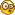' and then fires two or three rounds and im down......... Could it be thats were the intrusion is happening maybe, or is it maybe were the communication gets broken between my ps3 and their server and that intrusions is actually some port or something that is closed and my bullets is  never hitting the target at all on server side?Logged

#### FurryNutz

• Poweruser
•   ▲
▲ ▲
•• Posts: 49205
•##### Re: Do I need to worry about these...
« Reply #9 on: March 31, 2014, 07:50:15 AM »

• What Hardware version is your modem? Look at sticker under modem.
• Link>What Firmware version is currently loaded? Found on the modems web page under status.
• What region are you located?
• Are you wired or wireless connected to the modem?

Internet Service Provider and Modem Configurations
• What is the modem model # do you have?
• Is ISP Modem/Service using Dynamic or Static WAN IP addressing?
• What ISP Modem service link speeds UP and Down do you have?
• Check cable between Modem and Router, swap out to be sure. Link> Cat6 is recommended.
• Check ISP MTU requirements, Cable is usually 1500, DSL is around 1492 down to 1472. Call the ISP and ask. Link>Checking MTU Values
• For DSL/PPPoE connections on the router, ensure that "Always ON" option is enabled.

well said and thanx for putting my mind at ease!  Can not argue with a 'in ure face' fact now right!  But now for another noob question, if I may.  Is it normal for being so many kernel intrusions?  It usually happens when I'm online gaming with my ps3 playing BF4 and most of the times i battle to get ahead on the battlefield, sometimes i need to offload a hole clip, or most of it anyway, on a enemy and then he turns around 'look at me strangely' and then fires two or three rounds and im down......... Could it be thats were the intrusion is happening maybe, or is it maybe were the communication gets broken between my ps3 and their server and that intrusions is actually some port or something that is closed and my bullets is  never hitting the target at all on server side?Logged
"Nothing Funny about It...." We are not here to Impress anyone! You have a be a COMPETENT user first to under stand COMPETENT help!
Pages: 

BlackRain , 2006 by Crip